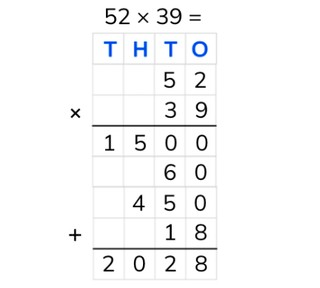Partial products algorithm with two numbers to 100

# Partial products algorithm with two numbers to 100

Students learn to multiply partial products with two numbers to 100.

No account needed.8,000 schools use Gynzy92,000 teachers use Gynzy1,600,000 students use Gynzy

## General

Students learn partial products multiplication with two numbers to 100.

## Standards

CCSS.Math.Content.4.NBT.B.5

## Learning objective

Students will be able to multiply partial products with two numbers to 100.

## Introduction

On the interactive whiteboard there is a multiplication problem and triangles with possible answers and more multiplication problems. Have the students solve the problem and drag the triangle with the correct answer to match up with the problem. Next the students solve the multiplication problem on that triangle. At the end the students must solve a problem, for which there are no more possible answers given.

## Instruction

Explain that with partial products multiplication you put the numbers one under the other. Next you can multiply the numbers by calculating from left to right. Explain that you put the largest number of the problem on top and the other number underneath that. With partial products multiplication you work from left to right. So you start by multiplying the tens numbers together. After that you multiply the lower tens number with the ones number on top. Next you multiply the lower right ones number with the upper left tens number. And then you multiply the ones numbers. You put all these outcomes in the chart and state the intermediate problems. If you add up the numbers in the chart, you get your answer. You put this answer at the bottom of the chart. Next there are multiplication problems in a chart, each one of which with a different row marked in yellow. Ask the students if they know which intermediate problem belongs with each of the colored rows. This is how you can check if the students know which steps they must take. After this you explain that if you have a problem in which you must multiply by 0, you don't have to write down the answer. When you multiply by 0 the answer is always 0. Next you show a chart, for which the intermediate steps must be filled in. Have the students write down these numbers and hold them up, so that you can check them. Then, practice partial products multiplication with two problems, of which one has the chart already filled in. The other chart still needs to be filled in.

Check whether the students can multiply with partial products by asking the following questions:
- Why is it useful to be able to multiply with partial products?
- What do the letters T H T O mean?
- Where do you always start when multiplying partial products?
- Solve this problem using partial products: 38 × 69

## Quiz

The students test their understanding of partial products algorithm with two numbers to 100 though ten exercises. For some of the exercises the students must fill in the intermediate steps, and for others they must calculate the answer. Some of the THTO-charts are filled in, and others the students must fill in on their own.

## Closing

You discuss with the students once again that it is important to be able to calculate partial products with two numbers to 100, because that is how you can calculate multiplication problems in steps. Check if the students know that with partial products multiplication they must multiply from left to right. Next the students must solve each other's problems using partial products.

## Teaching tips

When students have difficulty with partial products multiplication, you can repeat the meaning of T H T O and how you put the numbers in a THTO-chart. Write out the intermediate problems. You may also label the tens and the ones, so that it is clear what you are multiplying together.

### The online teaching platform for interactive whiteboards and displays in schools

• Save time building lessons

• Manage the classroom more efficiently

• Increase student engagement